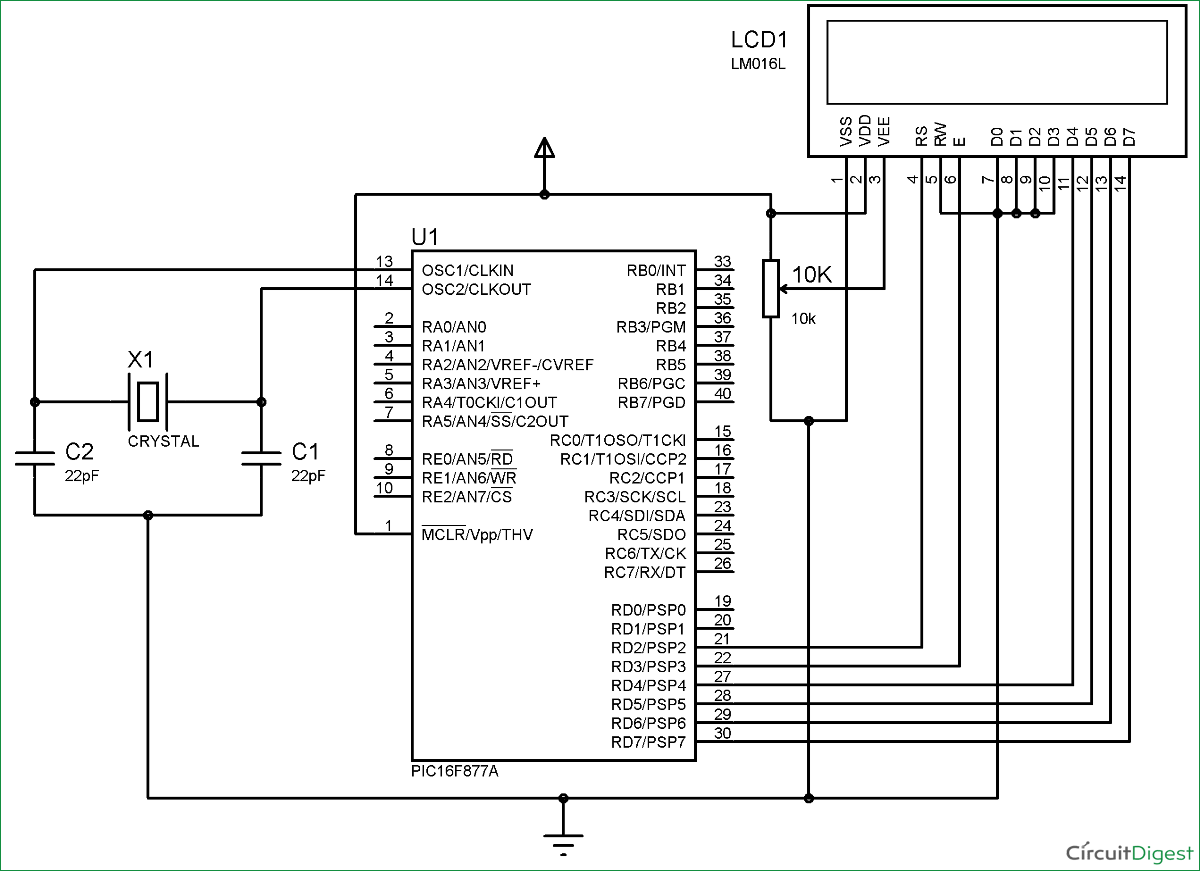# LCD Interfacing with PIC Microcontroller using MPLABX and XC8

This is our sixth tutorial in our PIC Tutorial Series, in this tutorial we learn Interfacing of 16×2 LCD with PIC Microcontroller. In our previous tutorials we have learnt the basics of PIC using some LED blinking Programs and have also learnt How to use Timers in PIC Microcontroller. You can check here all the tutorials on Learning PIC Microcontrollers using MPLABX and XC8 compiler.

This tutorial will be an interesting one because we will learn How to Interface 16×2 LCD with PIC16F877A, check the detailed Video at the end this tutorial. Gone are the old days where we used LEDs for user indications. Let us see how we can make our projects look more cool and useful by using LCD displays. Also check our previous articles on Interfacing LCD with 8051, with Arduino, with Raspberry Pi, with AVR.

### Functions for Interfacing LCD with PIC Microcontroller:

To make things easier we have made a small library that could make things easy while using this LCD with our PIC16F877A. The header file “MyLCD.h” is given here for download, which contains all the necessary function to drive the LCD using PIC MCU. Library code is well explained by comment lines but if you still have doubts reach us through the comment section. Also check this article for Basic LCD working and its Pinouts.

Note: It is always recommended to know what is actually happening inside your header file because it will help you in debugging or while changing the MCU.

Here I have copied and pasted the header file code into my main C file. So if you are using our code, then you don’t need to download and add the header file into your program, just use the complete Code given at the end of this Tutorial. Also note that this library will only support PIC16F series PIC Microcontroller.

Here I am explaining each function inside our header file below:

void Lcd_Start(): This function should be the first function that has to be called to start working with our LCD. We should call this function only once to avoid lag in the program.

```void Lcd_Start()
{
Lcd_SetBit(0x00);
for(int i=1065244; i<=0; i--)  NOP();
Lcd_Cmd(0x03);
__delay_ms(5);
Lcd_Cmd(0x03);
__delay_ms(11);
Lcd_Cmd(0x03);
Lcd_Cmd(0x02); //02H is used for Return home -> Clears the RAM and initializes the LCD
Lcd_Cmd(0x02); //02H is used for Return home -> Clears the RAM and initializes the LCD
Lcd_Cmd(0x08); //Select Row 1
Lcd_Cmd(0x00); //Clear Row 1 Display
Lcd_Cmd(0x0C); //Select Row 2
Lcd_Cmd(0x00); //Clear Row 2 Display
Lcd_Cmd(0x06);
}```

Lcd_Clear(): This function clears the LCD screen and can be used inside loops to clear the appearance of previous data.

```Lcd_Clear()
{
Lcd_Cmd(0); //Clear the LCD
Lcd_Cmd(1); //Move the cursor to first position
}```

void Lcd_Set_Cursor(x pos, y pos): Once started, our LCD is ready to take commands, we can instruct the LCD to set its cursor in you preferred location by using this function.  Suppose if, we need out cursor at 5th character of 1st row. Then the function will be void Lcd_Set_Cursor(1, 5)

```void Lcd_Set_Cursor(char a, char b)
{
char temp,z,y;
if(a== 1)
{
temp = 0x80 + b - 1; //80H is used to move the cursor
z = temp>>4; //Lower 8-bits
y = temp & 0x0F; //Upper 8-bits
Lcd_Cmd(z); //Set Row
Lcd_Cmd(y); //Set Column
}
else if(a== 2)
{
temp = 0xC0 + b - 1;
z = temp>>4; //Lower 8-bits
y = temp & 0x0F; //Upper 8-bits
Lcd_Cmd(z); //Set Row
Lcd_Cmd(y); //Set Column
}
}```

void Lcd_Print_Char(char data)  : Once the cursor is set we can write a character to its position by simple calling this function.

```void Lcd_Print_Char(char data)  //Send 8-bits through 4-bit mode
{
char Lower_Nibble,Upper_Nibble;
Lower_Nibble = data&0x0F;
Upper_Nibble = data&0xF0;
RS = 1;             // => RS = 1
Lcd_SetBit(Upper_Nibble>>4);             //Send upper half by shifting by 4
EN = 1;
for(int i=2130483; i<=0; i--)  NOP();
EN = 0;
Lcd_SetBit(Lower_Nibble); //Send Lower half
EN = 1;
for(int i=2130483; i<=0; i--)  NOP();
EN = 0;
}```

void Lcd_Print_String(char *a): If a group of characters is to be displayed, then the string function can be used.

```void Lcd_Print_String(char *a)
{
int i;
for(i=0;a[i]!='\0';i++)
Lcd_Print_Char(a[i]);  //Split the string using pointers and call the Char function
}```

Each time the Lcd_Print_Char(char data)  is called, its respective character values is sent to the data-lines of the LCD. These characters reach the HD44780U in form of bits. Now this IC relates the bits to the character to be displayed by using its ROM memory as shown the below table. You can find bits for all the characters in the datasheet of HD44780U LCD Controller.

### Circuit Diagram and Testing:

Below is the circuit diagram for Interfacing 16×2 LCD with PIC Microcontroller.For more detail: LCD Interfacing with PIC Microcontroller using MPLABX and XC8